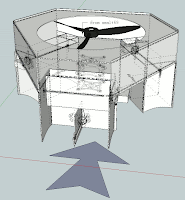### Pls help, how to change behavior of servo to like this?

Hi guys. I was building a monster, it's a proof model of fiying car. The Control System is like CCPM, but not exactly same.

I want change servo throws to half:This concept is the air one way in to three way out, 3 way out control flow rate by servo.Because the structure of my monster wasn't same as traditional helicopter, so control system need do some change.##If it's too small can't see, you can go there look bigger pic:

http://spoflycar.blogspot.com/p/control-system.html

I want know, How to change code to do servo throw limit half.  I tried set param of servo in "Mission Planner" but failed.

I am not good at coding, so can't found the place where the servo control. If you know this, please tell me, i want try change it. Thank you.

#### Replies

•Interesting...  yeah, you'll need some custom code to do this.  What you want is all within AP_MotorsHeli.cpp, which is in the AP_Motors library.

Specifically, these functions would be involved:

void AP_MotorsHeli::init_swash()
{

// swash servo initialisation
_servo_1->set_range(0,1000);
_servo_2->set_range(0,1000);
_servo_3->set_range(0,1000);
_servo_4->set_angle(4500);

// ensure _coll values are reasonable
if( collective_min >= collective_max ) {
collective_min = 1000;
collective_max = 2000;
}
collective_mid = constrain(collective_mid, collective_min, collective_max);

// calculate throttle mid point
throttle_mid = ((float)(collective_mid-collective_min))/((float)(collective_max-collective_min))*1000.0;

// determine roll, pitch and throttle scaling
_roll_scaler = (float)roll_max/4500.0;
_pitch_scaler = (float)pitch_max/4500.0;
_collective_scalar = ((float)(collective_max-collective_min))/1000.0;
_stab_throttle_scalar = ((float)(stab_col_max - stab_col_min))/100.0;

if( swash_type == AP_MOTORS_HELI_SWASH_CCPM ) { //CCPM Swashplate, perform control mixing

// roll factors
_rollFactor[CH_1] = cos(radians(servo1_pos + 90 - phase_angle));
_rollFactor[CH_2] = cos(radians(servo2_pos + 90 - phase_angle));
_rollFactor[CH_3] = cos(radians(servo3_pos + 90 - phase_angle));

// pitch factors

// collective factors
_collectiveFactor[CH_1] = 1;
_collectiveFactor[CH_2] = 1;
_collectiveFactor[CH_3] = 1;

}else{ //H1 Swashplate, keep servo outputs seperated

// roll factors
_rollFactor[CH_1] = 1;
_rollFactor[CH_2] = 0;
_rollFactor[CH_3] = 0;

// pitch factors
_pitchFactor[CH_1] = 0;
_pitchFactor[CH_2] = 1;
_pitchFactor[CH_3] = 0;

// collective factors
_collectiveFactor[CH_1] = 0;
_collectiveFactor[CH_2] = 0;
_collectiveFactor[CH_3] = 1;
}

// servo min/max values

// mark swash as initialised
_swash_initialised = true;
}

//
// heli_move_swash - moves swash plate to attitude of parameters passed in
// - expected ranges:
// roll : -4500 ~ 4500
// pitch: -4500 ~ 4500
// collective: 0 ~ 1000
// yaw: -4500 ~ 4500
//
void AP_MotorsHeli::move_swash(int16_t roll_out, int16_t pitch_out, int16_t coll_in, int16_t yaw_out)
{
int16_t yaw_offset = 0;
int16_t coll_out_scaled;

if( servo_manual == 1 ) { // are we in manual servo mode? (i.e. swash set-up mode)?
// check if we need to free up the swash
if( _swash_initialised ) {
reset_swash();
}
coll_out_scaled = coll_in * _collective_scalar + _rc_throttle->radio_min - 1000;
}else{ // regular flight mode

// check if we need to reinitialise the swash
if( !_swash_initialised ) {
init_swash();
}

// rescale roll_out and pitch-out into the min and max ranges to provide linear motion
// across the input range instead of stopping when the input hits the constrain value
// these calculations are based on an assumption of the user specified roll_max and pitch_max
// coming into this equation at 4500 or less, and based on the original assumption of the
// total _servo_x.servo_out range being -4500 to 4500.
roll_out = roll_out * _roll_scaler;
roll_out = constrain(roll_out, (int16_t)-roll_max, (int16_t)roll_max);

pitch_out = pitch_out * _pitch_scaler;
pitch_out = constrain(pitch_out, (int16_t)-pitch_max, (int16_t)pitch_max);

// scale collective pitch
coll_out = constrain(coll_in, 0, 1000);
if (stab_throttle){
coll_out = coll_out * _stab_throttle_scalar + stab_col_min*10;
}
coll_out_scaled = coll_out * _collective_scalar + collective_min - 1000;

// rudder feed forward based on collective
if( !ext_gyro_enabled ) {
yaw_offset = collective_yaw_effect * abs(coll_out_scaled - throttle_mid);
}
}

// swashplate servos
_servo_1->servo_out = (_rollFactor[CH_1] * roll_out + _pitchFactor[CH_1] * pitch_out)/10 + _collectiveFactor[CH_1] * coll_out_scaled + (_servo_1->radio_trim-1500);
_servo_2->servo_out = (_rollFactor[CH_2] * roll_out + _pitchFactor[CH_2] * pitch_out)/10 + _collectiveFactor[CH_2] * coll_out_scaled + (_servo_2->radio_trim-1500);
if( swash_type == AP_MOTORS_HELI_SWASH_H1 ) {
_servo_1->servo_out += 500;
_servo_2->servo_out += 500;
}
_servo_3->servo_out = (_rollFactor[CH_3] * roll_out + _pitchFactor[CH_3] * pitch_out)/10 + _collectiveFactor[CH_3] * coll_out_scaled + (_servo_3->radio_trim-1500);
_servo_4->servo_out = yaw_out + yaw_offset;

// use servo_out to calculate pwm_out and radio_out
_servo_1->calc_pwm();
_servo_2->calc_pwm();
_servo_3->calc_pwm();
_servo_4->calc_pwm();

// actually move the servos

// to be compatible with other frame types

// output gyro value
if( ext_gyro_enabled ) {
_rc->OutputCh(AP_MOTORS_HELI_EXT_GYRO, ext_gyro_gain);
}
}